# KCET 2019 Physics Paper with Solutions

KCET 2019 Physics paper with solutions are provided on this page. Students can view the question paper and analyze the solutions prepared by experts so that they can be familiar with the KCET exam pattern, question types, weightage of marks and basically understand the how the answer was formulated. This will also help students develop better problem-solving skills. Additionally, by going through the questions and the solutions they will get an idea about the difficulty level and the time it will take to solve this paper. The KCET question paper along with the solutions are available in a PDF format and can be download from this page.

### KCET 2019 - Physics

Question 1: Which one of the following nuclei has a shorter mean life?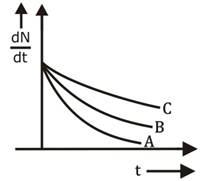1. a. B
2. b. Same for all
3. c. A
4. d. C

Solution:

Activity is given by the slope of the graph, higher activity implies a shorter mean life.

Slope of A is the greatest ⇒ higher activity ⇒ shorter mean life.

Question 2: The conductivity of a semiconductor increases with an increase in temperature because

1. a. Relaxation time increases
2. b. Number density of current carriers increases, relaxation time decreases but the effect of decrease in relaxation time is much less than the increase in number density
3. c. Number density of charge carriers increases
4. d. Both number density of charge carriers and relaxation time increase

Solution:

As temperature increases, the number density of charge carriers increases according to the equation

n = CT3/2e(-EKT/2)

Thus as temperature increases, the number density of charge carriers increases resulting in increased conductivity of semiconductors.

Question 3: For a transistor amplifier, the voltage gain

1. a. Is high at high and low frequencies and constant in the middle-frequency range
2. b. Constant at high frequencies and low at low frequencies
3. c. Remains constant for all frequencies
4. d. Is low at high and low frequencies and constant at mid frequencies

Solution:

The frequency response curve of the transistor amplifier shows that the voltage gain of a transistor amplifier is constant at mid-frequency range only. It is low at high and low frequencies.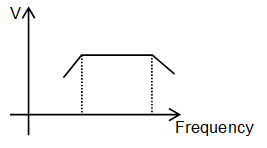Question 4: In the following circuit, what are P and Q?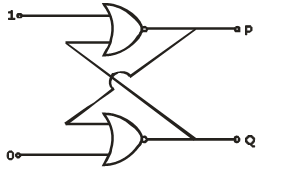1. a. P = 1, Q = 0
2. b. P = 1, Q =1
3. c. P = 0, Q = 0
4. d. P = 0, Q =1

Solution:

For both NOR gates

Output: Y = $\overrightarrow{A+B}$

For gate 1; A = 1

P = 0

For gate 2; B = 0

Q =1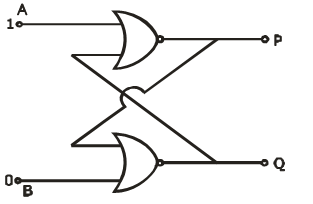Question 5: An antenna uses electromagnetic waves of frequency 5 MHz. For proper working, the size of the antenna should be

1. a. 300 m
2. b. 3 km
3. c. 15 m
4. d. 15 km

Solution:

C = f λ

λ = c/f

= 3×108/5×106

= (3/5)×102

= 60 m

Antenna length = λ/4 = 60/4 = 15 m

Question 6: A magnetic needle has a magnetic moment of 5×10-2 Am2 and moment of inertia 8×10-6 kgm2. It has a period of oscillation of 2s in a magnetic field $\vec{B}$. The magnitude of the magnetic field is approximately;

1. a. 0.4×10-4T
2. b. 0.8 ×10-4T
3. c. 1.6 ×10-4T
4. d. 3.2×10-4T

Solution:

T = 2π√(I/MB)

2 = 2π √(8×10-6)/(5×10-2×B)

⇒ B = 1.6×10-4 T

Question 7: A toroid has 500 turns per metre length. If it carries a current of 2A, the magnetic energy density inside the toroid is

1. a. 0.314 J/m3
2. b. 3.14 J/m3
3. c. 0.628 J/m3
4. d. 6.28 J/m3

Solution:

The magnetic field inside a toroid;

B = μ0NI

Magnetic energy density inside the toroid = B2/2μ0

Question 8: Consider the situation given in the figure. The wire AB is slid on the fixed rails with a constant velocity. If the wire AB is replaced by a semicircular wire, the magnitude of the induced current will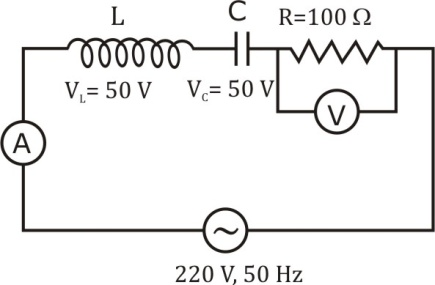1. a. Remain same
2. b. Increase or decrease depending on whether the semicircle bulges towards the resistance or away from it
3. c. Increase
4. d. Decrease

Solution:

Induced emf, ε = l . $(\vec{V}\times \vec{B})$

= (BV sinθ)l

= BVl

Induced current, I = ε/R

If the straight wire is replaced by a semicircular wire resistance remain the same, therefore, current also remains the same.

Question 9: The frequency of an alternating current is 50 Hz. What is the minimum time taken by current to reach its peak value from rms value?

1. a. 2.5×10-3s
2. b. 10×10-3s
3. c. 5×10-3s
4. d. 0.02 s

Solution:

Time period, T = 1/f = 1/50 sec

Time taken by current to reach peak value in Ac = T/4

⇒ T/4 = 1/(50×4) = 1/200

= 5×10-3 s

Question 10: The readings of ammeter and voltmeter in the following circuit are respectively1. a. 1.5 A, 100 V
2. b. 2.2 A, 220 V
3. c. 1.2 A, 120 V
4. d. 2.7 A, 220 V

Solution:

As evident from question

VL = VC

Circuit is purely resistive

V = 220 V

Also; I = V/R = 220/100

I = 2.2 A

Question 11: Two metal plates are separated by 2 cm. The potentials of the plates are -10 V, and + 30 V. The electric field between the two plates is

1. a. 1000V/m
2. b. 3000V/m
3. c. 500V/m
4. d. 2000V/m

Solution:

Electric field: E = V/d

V → Potential difference

d → separation between plates

E = 30-(-10)/2×10-2

E = 2000 V/m

Question 12: The equivalent capacitance between A and B is,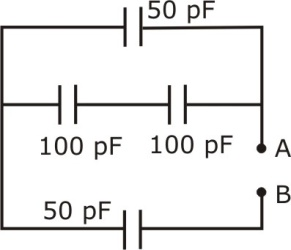1. a. 100/3 pF
2. b. 300 pF
3. c. 50 pF
4. d. 150 pF

Solution: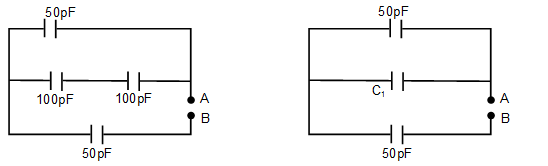C1 = (100×100)/(100+100)

= 50 pF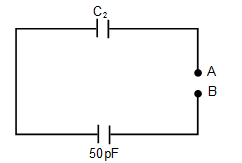C2 = 50 + C1

= 50+50

= 100pF

Now; C2 and 50pF are in series across AB.

CAB = (50×100)/(50+100)

= 5000/150

= 100/3 pF

Question 13: A capacitor of capacitance C charged by an amount Q is connected in parallel with an uncharged capacitor of capacitance 2C. The final charges on the capacitors are

1. a. Q/4, 3Q/4
2. b. Q/5, 4Q/5
3. c. Q/2, Q/2
4. d. Q/3, 2Q/3

Solution:

Let the common potential attained by the two capacitor be Vc

Vc = Total charge/Total capacitance = (Q+0)/(C+2C)

Vc = Q/3C

Final charge on capacitors

Q1 = CVc = CQ/3C = Q/3

Q2 = 2CVc = 2Q/3

Question 14: Though the electron drift velocity is small and the electron charge is very small, a conductor can carry an appreciably large current because

1. a. Drift velocity of the electron is very large
2. b. Relaxation time is small
3. c. Electron number density is very large
4. d. Electron number density depends on temperature

Solution:

Current: I = neAVd

The large value of I is due to the number density of free electron in a conductor (n), which is of the order of n = 1028 per m3.

Question 15: Masses of three wires of copper are in the ratio 1:3:5 and their lengths are in the ratio 5:3:1. The ratio of their electrical resistance is

1. a. 5:3:1
2. b. 125:15:1
3. c. 1:3:5
4. d. 1:15:125

Solution:

Resistance of wire: R = ρL/A

ρ → resistivity of wire

Area: A = Volume/Length = V/L

Volume = Mass/Density = m/d

R = ρdL2/m

R ∝ L2/m

R1:R2: R3 = L12/m1 : L22/m2: L32/m3

= 52/1: 32/3:12/5

= 125:15:1

Question 16: If P, Q and R are physical quantities having different dimensions, which of the following combinations can never be a meaningful quantity?

1. a. PQ-R
2. b. (PR-Q2)/R
3. c. (P-Q)/R
4. d. PQ/R

Solution:

By the principle of Homogeneity; Addition & subtraction of physical quantities having different dimensions is not possible.

Question 17: The given graph shows the variation of velocity (v) with position (x) for a particle moving along a straight line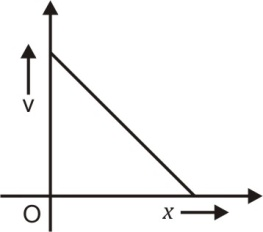Which of the following graph shows the variation of acceleration (a) with position (x)?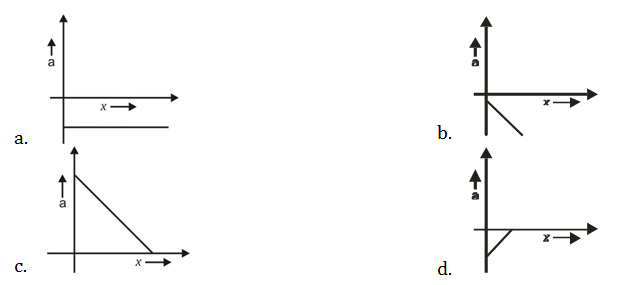1. a.= -m(-mx+V0) a.= +m2x-mV0….(2)

Slope for given eq. (2): +Ve

Intercept for eq. (2):–Ve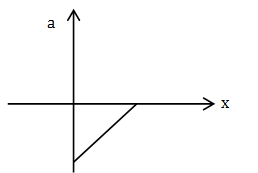Solution:

The equation for the given graph can be written as.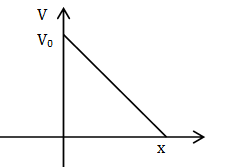V = -mx+v0 ..(1)

m slope of graph which is -ve

V0 intercept of line which is +V and a constant

a.= dV/dt = -m(dx/dt)+(d/dt)V0

a.= -mV+0

But from eqn (1)

a.= -m(-mx+V0) a.= +m2x-mV0….(2)

Slope for given eq. (2): +Ve

Intercept for eq. (2):–VeQuestion 18: The trajectory of a projectile projected from the origin is given by the equation y = x-2x2/5 . The initial velocity of the projectile is?

1. a. 5 ms-1
2. b. 5/2 ms-1
3. c. 2/5 ms-1
4. d. 25 ms-1

Solution:

Given; y = x-2x2/5

Comparing with standard equation of trajectory

i.e. y = x tan θ - (gx2/2u2cos2 θ)

tan θ = 1

⇒ θ = 45º

Also (g/2u2cos2 θ ) = 2/5

⇒ (g/2u2×(1/2)) = 2/5

⇒ u = 5m/s

Question 19: An object with mass 5 kg is acted upon by a force, $\vec{F}= -3\hat{i}+4\hat{j}$ N. If it’s initial velocity at t = 0 is $\vec{v}= 6\hat{i}-2\hat{j}$ ms-1, the time at which it will just have a velocity along y-axis is

1. a. 10 s
2. b. 15 s
3. c. 5 s
4. d. 2 s

Solution:

$\vec{F}= -3\hat{i}+4\hat{j}$

$a= \frac{\vec{F}}{m}$

= $\frac{-3}{5}\hat{i}+\frac{4}{5}\hat{j}$

ax = -3/5

At t = 0; V0 = $6\hat{i}-12\hat{j}$

Vat t=0 = 6

For body to have velocity only along y-axis, velocity along x-axis should be zero

Vx = Vox+axt

0 = 6-(3/5)t

t = 10 sec

Question 20: During inelastic collision between two objects, which of the following quantity always remains conserved?

1. a. Total mechanical energy
2. b. Speed of each body
3. c. Total kinetic energy
4. d. Total linear momentum

Solution:

Linear momentum always remains conserved for any type of collision.

Question 21: In Rutherford experiment, for a head-on collision of α -particles with a gold nucleus, the impact parameter is

1. a. of the order of 10-14 m
2. b. of the order of 10-6 m
3. c. zero
4. d. of the order of 10-10 m

Solution:

For head-on collision, the impact parameter is zero as it retraces its path.

Question 21: In Rutherford experiment, for a head-on collision of α -particles with a gold nucleus, the impact parameter is

1. a. of the order of 10-14 m
2. b. of the order of 10-6 m
3. c. zero
4. d. of the order of 10-10 m

Solution:

For head-on collision, the impact parameter is zero as it retraces its path.

Question 22: Frequency of revolution of an electron revolving in nth orbit of H-atom is proportional to

1. a. n
2. b. 1/n3
3. c. 1/n2
4. d. n independent of n

Solution:

Time period of revolution; T = 2 πr/V

Frequency; f = V/2 πr

Also V ∝ 1/n

And r ∝ n2 (By Bohr’s model)

f ∝ 1/n3

Question 23: A hydrogen atom in ground state absorbs 10.2 eV of energy. The orbital angular momentum of the electron is increased by

1. a. 2.11×10-34 Js
2. b. 4.22×10-34 Js
3. c. 1.05×10-34 Js
4. d. 3.16×10-34 Js

Solution:

By absorbing 10.2 eV electron goes to 2nd orbit, ie n = 2

Angular momentum increased by

L2-L1 = (2h-h)/2π

= h/2π

= 6.6×10-34/(2×3.14)

= 1.05×10-34 Js

Question 24: The end product of decay of 90Th232 is 82Pb208. The number of α and β particles emitted are respectively.

1. a. 6, 4
2. b. 4, 6
3. c. 3, 3
4. d. 6, 0

Solution: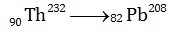Hence, mass number changes by 24 this implies 6 α particles are emitted as 1 α particle emitted decreases the mass number by 4, then proton number should decrease by 12, but the change in proton number is by 8 only, this implies 4 β particles have been emitted.

Question 25: Two protons are kept at a separation of 10 nm. Let Fn and Fe be the nuclear force and the electromagnetic force between them

a. Fe>>Fn

1. a. Fe>>Fn
2. b. Fe and Fn differ only slightly
3. c. Fe = Fn
4. d. Fe<<Fn

Solution:

As the separation is less than 10nm, therefore, Fe>>Fn as nuclear force is short-range force.

Question 26: Two particles which are initially at rest move towards each other under the action of their mutual attraction. If their speeds are v and 2v at any instant, then the speed of the centre of mass of the system is,

1. a. Zero
2. b. v
3. c. 2v
4. d. 1.5 v

Solution:

Initially both A and B are at rest, hence initial momentum is zero, Also no external force is acting on the two particles as they are moving under mutual attraction so final momentum should also be zero, by conservation of linear momentum.

Vcom = 0, as mass cannot be zero.

Question 27:A particle is moving uniformly along a straight line as shown in the figure. During the motion of the particle from A to B, the angular momentum of the particle about 'O'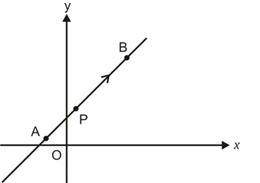1. a. Decreases
2. b. First increases then decreases
3. c. Increases
4. d. Remains constant

Solution:

Angular momentum = mvr

Here r is the perpendicular distance of particle from reference point i.e. O, so as m, v, r are constant for position A and B.

So angular momentum remains constant.

Question 28: A satellite is orbiting close to the earth and has a kinetic energy K. The minimum extra kinetic energy required by it to just overcome the gravitation pull of the earth is

1. a. 2K
2. b. 2√2
3. c. K
4. d. √3K

Solution:

We known that escape velocity

Ve = √2V0

V0 = orbital velocity

When satellite is in orbit; K = (½)mv02

Kinetic energy required to escape; K’ = (½)mve2 = mv02 = 2 K

Extra Kinetic energy = 2K – K = K

Question 29: A wire is stretched such that its volume remains constant. The Poisson's ratio of the material of the wire is

1. a. -0.50
2. b. -0.25
3. c. 0.50
4. d. 0.25

Solution:

Let L be the length and r be the radius of wire.

So Volume: V = πr2L

Differentiating both sides

∆V = π(2r∆r)L+ πr2∆L

As volume remains unchanged , ∆L = 0

0 = 2πrL∆r + πr2∆L

(∆r/r)/(∆L/L) = -1/2

Poisson’s ratio = Lateral strain/ longitudinal strain

= -(∆r/r)/(∆L/L)

= 1/2

= 0.5

Question 30: A cylindrical container containing water has a small hole at height of H = 8 cm from the bottom and at a depth of 2 cm from the top surface of the liquid. The maximum horizontal distance travelled by the water before it hits the ground (x) is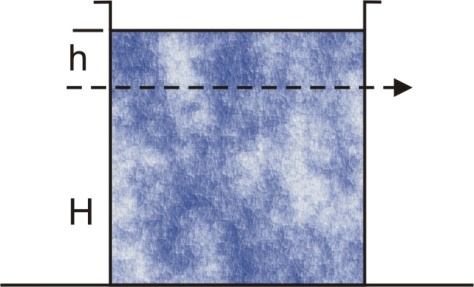1. a. 4√2 cm
2. b. 6 cm
3. c. 8 cm
4. d. 4 cm

Solution:

Vx = √(2gh)

h = 2×10-2

Vx = √(2×10×2×10-2)

= √(4/10)

= √(2/5

H = 8×10-2m

g = 10m/s2

∴ t = √(2H/g)

= √(2×8×10-2/10)

= 2/5√10 sec

Maximum horizontal distance = v×t

= √(2/5)× 2/5√10

= 2√2/5√50

= 2/25m

= 8 cm

Question 31: A transparent medium shows relation between i and r as shown. If the speed of light in vaccum is c, the Brewster angle for the medium is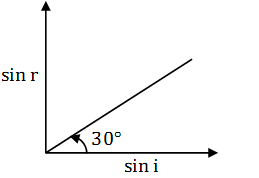1. a. 450
2. b. 900
3. c. 300
4. d. 600

Solution:

By Brewster’s law, n = tan θp

Also; n = sin i/sin r

But sin i/sin r = 1/slope

n = sin i/sin r = 1/tan 300 = √3

n = tan θp

= √3

θp = tan-1√3

θp = 600

Question 32: In Young’s double slit experiment, using monochromatic light of wavelength λ, the intensity of light at a point on the screen where path difference is λ is K units. The intensity of light at a point where path difference is λ/3 is

1. a. K/4
2. b. 2K
3. c. K
4. d. 4K

Solution:

We know that ∆x = (λ/2π) ∆ φ

I = K cos2 (2π/3)

I = K(-1/2)2

I = K/4

Intensity, I = I0 cos2 φ

I = K cos2 (2 π/3)

= K(-1/2)2

= K/4

Question 33: Due to Doppler's effect, the shift in wavelength observed is 0.1 Å for a star producing wavelength 6000 Å. Velocity of recession of the star will be

1. a. 10 km/s
2. b. 20 km/s
3. c. 25 km/s
4. d. 5 km/s

Solution:

Doppler shift: ∆ λ/ λ = V/C

V = (∆ λ/ λ)C

V = (0.1/6000)×3×108

V = 5km/s

Question 34: An electron is moving with an initial velocity $\vec{V}= V_{0}\hat{i}$ and is in a uniform magnetic field $\vec{B}= B_{0}\hat{j}$ . Then, it’s de Broglie wavelength

1. a. Increases with time
2. b. Increases and decreases periodically
3. c. Remains constant
4. d. Decreases with time

Solution:

Magnetic force on moving electron:

F = $-e (V_{0}\hat{i}\times B_{0}\hat{j})$

F = $-e V_{0} B_{0}\hat{k}$

So, Force is perpendicular to both $\vec{V} and \vec{B}$ so the magnitude of V will not change it means momentum will not change.

Now; by De- Broglie

λ = h/mv = h/p

As momentum remains constant.

So De Broglie wavelength remains constant.

Question 35: Light of certain frequency and intensity incident on a photosensitive material causes the photoelectric effect. If both the frequency and intensity are doubled, the photoelectric saturation current becomes.

1. a. doubled
2. b. unchanged
4. d. halved

Solution:

We know that photoelectric current is directly proportional to the intensity of light, given that incident frequency is greater than the threshold frequency.

Hence when intensity is doubled, photoelectric saturation current doubles.

Question 36: A certain charge 2Q is divided at first into two parts q1 and q2. Later, the charges are placed at a certain distance. If the force of interaction between two charges is maximum then Q/Q1 = __.

1. a. 2
2. b. 0.5
3. c. 4
4. d. 1

Solution:

Force of interaction between two charges q1 and q2 will be maximum when

q1 = q2 = 2Q/2 = Q

Q/q1 = Q/Q = 1

Question 37: A particle of mass m and charge q is placed at rest in uniform electric field E and then released. The kinetic energy attained by the particle after moving a distance y is

1. a. qE2y
2. b. q2Ey
3. c. qEy2
4. d. qEy

Solution:

Velocity gained after moving a distance y:

V2 = u2+2ay

Here; u = 0,

F = qE

ma = qE

a = qE/m

V2 = 0+2(qE/m)y

Kinetic energy, E = (1/2) mv2

E = (½)×m×2×(qE/m)×y

= qEy

Question 38: An electric dipole is kept in a non-uniform electric field. It generally experiences

1. a. A force but not a torque
2. b. Neither a force nor a torque
3. c. A force and torque
4. d. A torque but not a force

Solution:

An electric dipole in non-uniform electric field generally experiences a force and a torque.

Question 39: The figure gives the electric potential V as a function of distance through four regions on x-axis. Which of the following is true for the magnitude of the electric field E in these regions?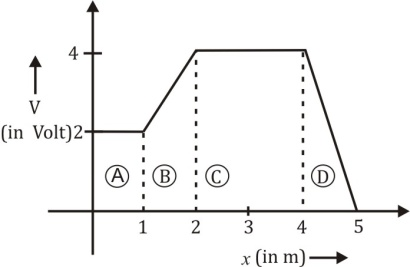1. a. EA = EC and EB< ED
2. b. EA< EB< EC< ED
3. c. EA> EB> EC> ED
4. d. EB = ED and EA< EC

Solution:

In region A, V is constant

EA = 0 as E = dV/dx

In region B, EB = (4-2)/2 = 1V/m

EB = 1V/m

In region C, V is constant

EC = 0

In region D, ED = (4-2)/1 = 2V/m

ED = 2V/M

So EA = EC and EB< ED

Question 40: A system of two charges separated by a certain distance apart stores electrical potential energy. If the distance between them is increased, the potential energy of the system,

1. a. Decreases in any case
2. b. Remains the same
3. c. Increases in any case
4. d. May increase or decrease

Solution:

Potential energy of system of 2 charges

U = $\frac{1}{4\pi \epsilon _{0}}\frac{q_{1}q_{2}}{r}$

r is separation between 2 charges q1 and q2.

If both charges are of the same type, then on increasing the separation, the potential energy decreases.

If the charges are of the opposite type, then on increasing separation, potential energy increases.

Question 41: In a cyclotron, a charged particle

1. a. Speeds up between the dees because of the magnetic field
2. b. Slows down within a dee and speeds up between dees
3. c. Undergoes acceleration all the time
4. d. Speeds up in dee

Solution:

In a cyclotron a charged particle experiences electric force between the dees and a magnetic force inside the dees while circulating, hence it always experiences a force and hence acceleration.

Question 42: The number of turns in a coil of Galvanometer is tripled, then

1. a. Voltage sensitivity remains constant and current sensitivity increases 3 times
2. b. Both voltage and current sensitivity decreases by 33%
3. c. Voltage sensitivity increases 3 times and current sensitivity remains constant
4. d. Both voltage and current sensitivity remains constant

Solution:

Current sensitivity: $S_{I}=\frac{\phi }{I}=\frac{NBA}{K}$

Voltage sensitivity: $S_{V}=\frac{\phi }{V}=\frac{NIBA}{KV}= \frac{NBA}{KR}$

When we triple “N” then R is also tripled

Hence $\frac{\phi }{I}\rightarrow$ becomes 3 times.

But $\frac{\phi }{V}\rightarrow$ remain unchanged

Question 43: A circular current loop of magnetic moment M is in an arbitrary orientation in an external uniform magnetic field $\vec{B}$. The work done to rotate the loop by 300 about an axis perpendicular to its plane is

1. a. √3MB/2
2. b. zero
3. c. MB
4. d. MB/2

Solution:

Even when the coil is rotated, about an axis perpendicular to its plane, the potential energy does not change. Hence, work done is zero

Question 44: In a permanent magnet at room temperature

1. a. The individual molecules have a nonzero magnetic moment which are all perfectly aligned

2. b. Domains are all perfectly aligned

3. c. Magnetic moment of each molecule is zero

4. d. Domains are partially aligned

Solution:

Even in case of permanent magnets all the domain are not perfectly aligned due to thermal agitation.

Question 45: Coersivity of a magnet where the ferromagnet gets completely demagnetized is 3 ×103 Am-1. The minimum current required to be passed in a solenoid having 1000 turns per metre, so that the magnet gets completely demagnetized when placed inside the solenoid is

1. a. 60 mA
2. b. 6A
3. c. 30 mA
4. d. 3A

Solution:

Coercivity refers to the intensity of magnetic field (B) where the magnets get demagnetized

For solenoid; B = μ0nI

n = No of turns per unit length

I = current through each turn

μ0 = Absolute permeability

B = magnetic field in solenoid

Also μ0 H = μ0nI

H = intensity of magnetic field

I = H/n

I = 3×103/(100/0.1) = 3A

Question 46: An inductor of inductance L and resistor R are joined together in series and connected by a source of frequency ω. The power dissipated in the circuit is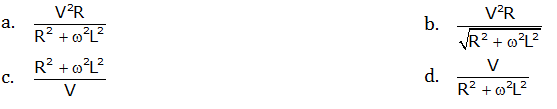Solution: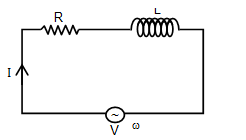Z = √(R2+XL2)

Z = √(R2+(ωL)2)

P = I2R

I = V/√(R22L2)

P = V/(R2+ ω2L2)

Question 47: An electromagnetic wave is travelling in x-direction with electric field vector given by $\vec{E_{y}}= E_{0}\sin (kx-\omega t)\hat{j}$. The correct expression for magnetic field vector is

1. a. $\vec{B_{z}}= E_{0}C\sin (kx-\omega t)\hat{k}$
2. b. $\vec{B_{z}}= \frac{E_{0}}{C}\sin (kx-\omega t)\hat{k}$
3. c. $\vec{B_{y}}= E_{0}C\sin (kx-\omega t)\hat{j}$
4. d. $\vec{B_{y}}= \frac{E_{0}}{C}\sin (kx-\omega t)\hat{j}$

Solution:

$\vec{E_{y}}= E_{0}\sin (kx-\omega t)\hat{j}$

C = E0/B0

B0 = E0/C

$\vec{B_{z}}= \frac{E_{0}}{C}\sin (kx-\omega t)\hat{k}$

Question 48: The phenomenon involved in the reflection of radio-waves by ionosphere is similar to

1. a. Total internal reflection of light in the air during a mirage
2. b. Scattering of light by air particles
3. c. Reflection of light by plane mirror
4. d. Dispersion of light by water molecules during the formation of a rainbow

Solution:

The phenomenon involved in the reflection of radio-waves by ionosphere is similar to total internal reflection of light in the air during a mirage.

Question 49: A point object is moving uniformly towards the pole of a concave mirror of focal length 25 cm along its axis as shown below. The speed of the object is 1ms-1. At t = 0, the distance of the object from the mirror is 50 cm. The average velocity of the image formed by the mirror between time t = 0 and t = 0.25 s is: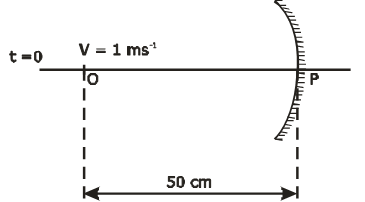1. a. 20 cm s-1
2. b. Infinity
3. c. 40 cm s-1
4. d. Zero

Solution:

Focal length = 2.5cm

At t = 0, object is at a distance of xi =50cm

At t = 0.25 object is at a distance of xf = speed × time

=0.1 × 0.25

= 0.25 m

= 25 cm

At t = 0.25 image will beat infinity

Vavg = ∆x/ ∆t

= ∞

Question 50: A certain prism is found to produce a minimum deviation of 380. It produces a deviation of 440 when the angle of incidence is either 420 or 620. What is the angle of incidence when it is undergoing minimum deviation?

1. a. 400
2. b. 600
3. c. 300
4. d. 490

Solution:

$\delta _{min}$ = 380

i = $\frac{A+\delta }{2}$

$\delta$ = deviation

i = angle of incidence

A = Angle of prism

$\delta$ = (r1+ r2)-A

-A = 44-104

A = 600

i = (60+38)/2 = 49

Question 51: In the given circuit, the current through 2 ohm resistor is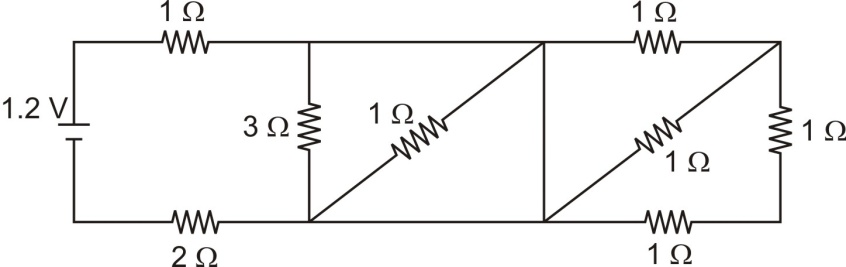1. a. 0.3A
2. b. 0.1A
3. c. 0.2A
4. d. 0.4A

Solution:

The circuit can be redrawn as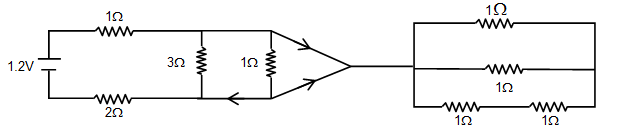Req = 1+2 = 3 ohm

I = V/Req = 1.2/3

= 0.4 A

Question 52: Kirchhoff's junction rule is a reflection of

1. a. Conservation of energy
2. b. Conservation of charges
3. c. Conservation of current density vector
4. d. Conservation of momentum

Solution:

Kirchhoff's junction rule is based on conservation of charges.

Question 53: The variation of terminal potential difference (V) with current flowing through a cell is as shown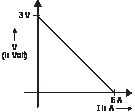The emf and internal resistance of the cell are

1. a. 3V, 0.5 ohm
2. b. 6V, 0.5 ohm
3. c. 3V,2 ohm
4. d. 6V,2 ohm

Solution:

From the given graph, we can form the following equation

V = E-Ir

When I = 0,

E = V = 3V

When V = 0,

I = 6A

r = E/I

= 3/6

= 0.5 ohm.

Question 54: In a potentiometer experiment, the balancing point with a cell is at a length 240 cm. On shunting the cell with a resistance of 2 Ω, the balancing length becomes 120 cm. The internal resistance of the cell is

1. a. 2 Ω
2. b. 0.5 Ω
3. c. 4 Ω
4. d. 1 Ω

Solution:

Internal resistance of a cell is given by:

r = R((l1/l2)-1)

r = 2((240/120)-1)

r = 2×1

r = 2 Ω

Question 55: The magnetic field at the centre 'O' in the given figure is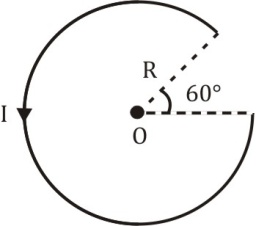1. a. 5μ0I/12 R
2. b. μ0I/12 R
3. c. 7μ0I/14 R
4. d. 3μ0I/10 R

Solution:

Here θ = 3000 as can be seen from given diagram

So number of turns, n = 300/360 = 5/6

B = μ0nI/2R

= μ0×(5/6)×(I/2R)

= 5μ0I/12 R

Question 56: An aluminium sphere is dipped into the water. Which of the following is true?

1. a. Buoyancy will be more in water at 00C than that in water at 40C
2. b. Buoyancy may be more or less in water at 40C depending on the radius of the sphere
3. c. Buoyancy will be less in water at 00C than that in water at 40 C
4. d. Buoyancy in water at 00C will be same as that in water at 40C

Solution:

Buoyancy at 00C; FB = Vρ0g

Buoyancy at 40C; FB’ = Vρ4g

FB/FB’ = ρ0/ ρ4

As we know at 40C is more than at 00C

ρ4> ρ0

FB < FB

Question 57: A thermodynamic system undergoes a cyclic process ABC as shown in the diagram. The work done by the system per cycle is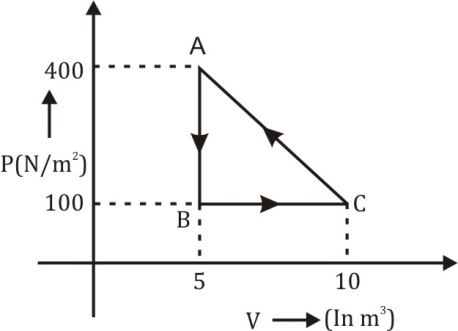1. a. -1250 J
2. b. 1250 J
3. c. 750 J
4. d. -750 J

Solution:

Work done = P ∆V

Also; work done = area of ∆ABC

W = (½) ×(10-5)×(100-400)

W = -750 J

Question 58: One mole of O2 gas is heated at constant pressure starting at 27 0C. How much energy must be added to the gas as heat to double it’s volume?

1. a. 450 R
2. b. 1050 R
3. c. Zero
4. d. 750 R

Solution:

If at constant pressure, the volume is doubled then the temperature also gets doubled.

T1 = 300K, T2 = 600 K

∆T = 600-300 = 300 K

Q = nCp∆T = 1×(7/2)R×300

= 1050 R

Question 59: A piston is performing S.H.M. in the vertical direction with a frequency of 0.5 Hz. A block of 10 kg is placed on the piston. The maximum amplitude of the system such that the block remains in contact with the piston is

1. a. 0.5 m
2. b. 0.1 m
3. c. 1 m
4. d. 1.5 m

Solution:

f = 0.5 Hz

ω = 2πf

= 2×π×(1/2)

= π

For block to remain in contact with the piston, at amplitude position of SHM

Weight of block = force due to oscillation

mg = ma

mg = m(ω2A)

A = g/ ω2

= 10/π2

So A ≈ 1m

Question 60: The equation of a stationary wave is $y=2\sin (\frac{\pi x}{15})\cos (48\pi t)$. The distance between a node and it’s next antinode is

1. a. 1.5 units
2. b. 30 units
3. c. 7.5 units
4. d. 22.5 units

Solution:

$y=2\sin (\frac{\pi x}{15})\cos (48\pi t)$

$\frac{2\pi }{\lambda }= \frac{\pi }{15}$

$\lambda =30$

So distance between node and next antinodes;

$\frac{\lambda }{4}=\frac{30}{4}= 7.5$

### KCET 2019 Physics Question Paper with Solutions